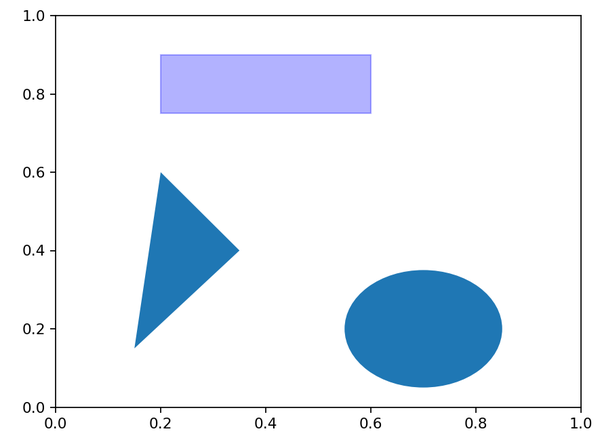# patch

```fig = plt.figure()

# 注意下面三种形状的参数提供方式
rect = plt.Rectangle((0.2,0.75),0.4,0.15,alpha=0.3,color='b')
circ = plt.Circle((0.7,0.2), 0.15,)
pgon = plt.Polygon([[0.15,0.15],[0.35,0.4],[0.2,0.6]])